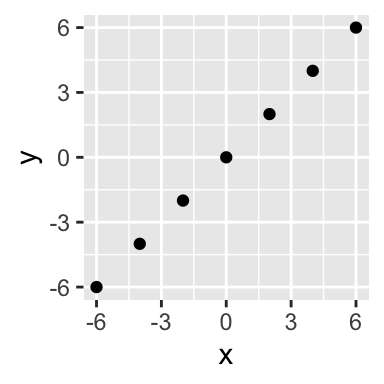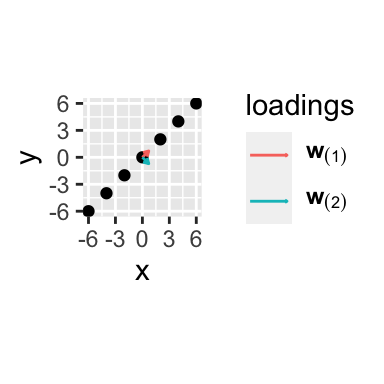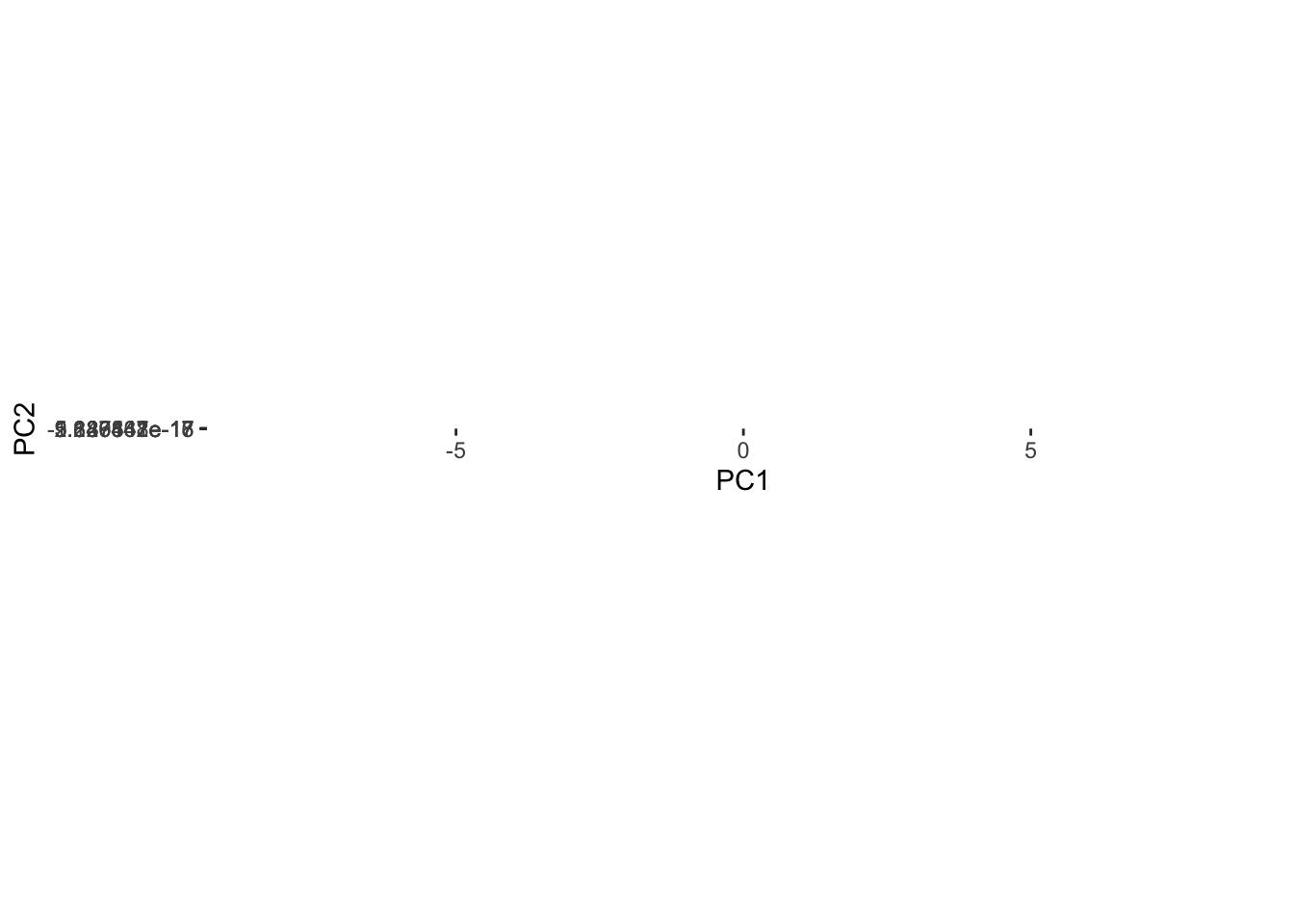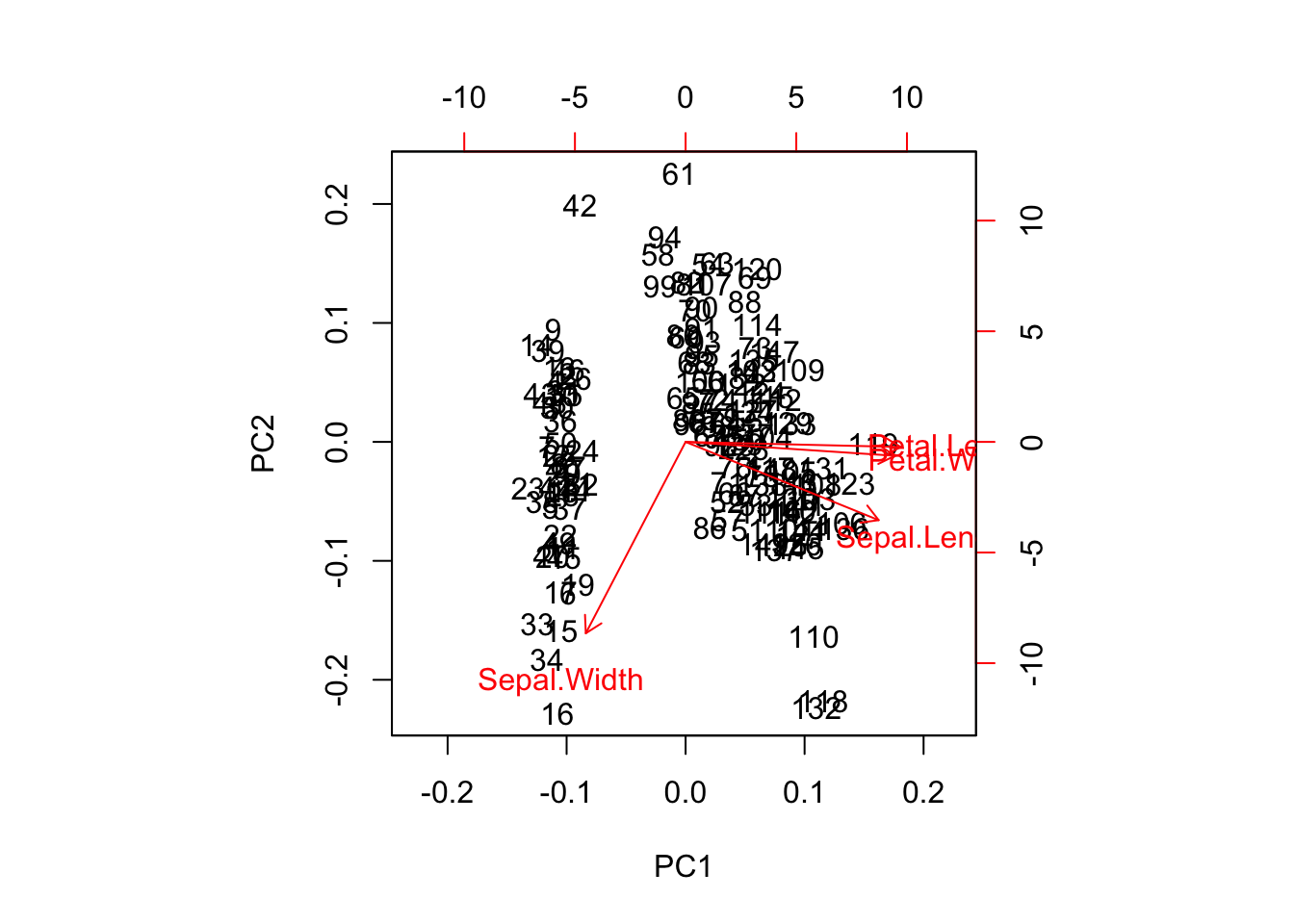## Theory

### Data matrix

I have a data matrix $$X$$ with $$n$$ rows (number of observations) and $$p$$ columns (number of features) with column-wise zero empirical mean. We don’ have response variable $$Y$$.

Each row of $$X$$ (observation), $$\vec{x}_{(i)} = (x_1, \dots, x_p)_{(i)}$$, is a vector in a $$p$$-dimensional space.

### Definition of PCA

PCA is defined as an orthogonal linear transformation of the data to a new coordinate system such that

• the first coordinate (called the first principal component) is the coordinate for which the variance of the projection of the observations into the axis is the greatest

• the second coordinate (called the second principal component) is the coordinate for which the variance of the projection of the observations into the axis is the second greatest

• and so on.

### The new coordinate system

The new coordinate system is defined by the vectors of weights or loadings

$\vec{w}_{(k)} = (w_1, \dots, w_p)_{(k)} \quad \text{for} \quad k=1 \dots m$

### Vector of principal components scores

The vector of principal components scores, $$\vec{t}_{(i)} = (t_1, \dots, t_m)_{(i)}$$, is the map of the vector $$\vec{x}_{(i)}$$ in the new coordinate system.

For a given observation $$\vec{x}_{(i)}$$, the $$k$$ score is given by the projection of $$\vec{x}_{(i)}$$ over $$\vec{w}_{(k)}$$

$t_{k(i)} = \vec{x}_{(i)} \cdot \vec{w}_{(k)} \quad \text{for} \quad i=1 \dots n \qquad k=1 \dots m$

### Matrix notation

If $$W$$ is a matrix in which column $$k$$ is $$\vec{w}_{(k)}$$ and $$T$$ is matrix in which each row is $$t_{(i)}$$, then

$T = X \cdot W$

It could be demonstrated that the loadings are the eigenvectors of $$X^T X$$ ordered from decreasing value of the eigenvalue.

### Simple example

We create a data matrix

library(tidyverse)

set.seed(40)
X <- tibble(x = seq(-6, 6, 2), y = x) %>%
rowwise() %>%
mutate(x = x + rnorm(1), y = y + rnorm(1)) %>%
ungroup() %>%
mutate(x = x - mean(x), y = y - mean(y)) %>%
as.matrix()

dim(X)
##  7 2
X
##               x             y
## 1 -6.000000e+00 -6.000000e+00
## 2 -4.000000e+00 -4.000000e+00
## 3 -2.000000e+00 -2.000000e+00
## 4  5.551115e-17 -1.110223e-16
## 5  2.000000e+00  2.000000e+00
## 6  4.000000e+00  4.000000e+00
## 7  6.000000e+00  6.000000e+00
p <- ggplot(X %>% as_tibble(), aes(x,y)) +
geom_point() +
coord_equal()
pWe use prcomp to calculate the loadings

pr <- prcomp(X, center = FALSE, scale = FALSE)

w <- pr$rotation w_plot <- as_tibble(t(w)) %>% bind_cols(tibble(component = rownames(t(w)))) p + geom_segment(data = w_plot, aes(x = 0, y = 0, xend = x, yend = y, color = component), arrow = arrow(length = unit(0.05, "npc"))) + scale_color_discrete(name = "loadings", breaks = c("PC1", "PC2"), labels = c(expression(bold(w)[(1)]), expression(bold(w)[(2)]))) + coord_equal() ## Coordinate system already present. Adding new coordinate system, which will replace the existing one.We calculate the principal components scores using the loadings X %*% w ## PC1 PC2 ## 1 -8.485281e+00 0.000000e+00 ## 2 -5.656854e+00 0.000000e+00 ## 3 -2.828427e+00 0.000000e+00 ## 4 -3.925231e-17 1.177569e-16 ## 5 2.828427e+00 0.000000e+00 ## 6 5.656854e+00 0.000000e+00 ## 7 8.485281e+00 0.000000e+00 and check that corresponds to the principal components scores given directly by prcomp pr$x
##             PC1          PC2
## 1 -8.485281e+00 0.000000e+00
## 2 -5.656854e+00 0.000000e+00
## 3 -2.828427e+00 0.000000e+00
## 4 -3.925231e-17 1.177569e-16
## 5  2.828427e+00 0.000000e+00
## 6  5.656854e+00 0.000000e+00
## 7  8.485281e+00 0.000000e+00
X %*% w == pr$x ## PC1 PC2 ## 1 TRUE TRUE ## 2 TRUE TRUE ## 3 TRUE TRUE ## 4 TRUE TRUE ## 5 TRUE TRUE ## 6 TRUE TRUE ## 7 TRUE TRUE We plot the the scores in the new coordinate system ggplot(pr$x %>% as_tibble(), aes(PC1, PC2)) +
geom_point() +
coord_equal()## Checking centering and scaling in prcomp

library(tidyverse)

iris_center_scale <- iris %>%
mutate_if(is.numeric, funs( (. - mean(.)) / sd(.)))
## Warning: funs() is soft deprecated as of dplyr 0.8.0
## Please use a list of either functions or lambdas:
##
##   # Simple named list:
##   list(mean = mean, median = median)
##
##   # Auto named with tibble::lst():
##   tibble::lst(mean, median)
##
##   # Using lambdas
##   list(~ mean(., trim = .2), ~ median(., na.rm = TRUE))
## This warning is displayed once per session.
# we check that is properly centered and scaled
iris_center_scale %>% summarise_if(is.numeric, funs(mean, sd))
##   Sepal.Length_mean Sepal.Width_mean Petal.Length_mean Petal.Width_mean
## 1     -4.484318e-16     2.034094e-16     -2.895326e-17    -2.989362e-17
##   Sepal.Length_sd Sepal.Width_sd Petal.Length_sd Petal.Width_sd
## 1               1              1               1              1

PCA is the centered and scaled data

prcomp(iris_center_scale[-5], scale = FALSE, center = FALSE) %>%
biplot()PCA automatically centering and scaling

prcomp(iris[-5], scale = TRUE, center = TRUE) %>%
biplot()